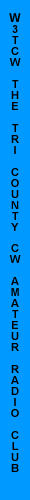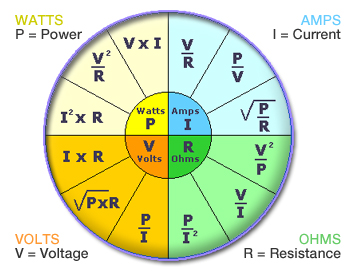THE TRI-COUNTY CW AMATEUR RADIO CLUBACTIVITIES / NEWS

Western Pennsylvania Section Emergency Response Plan

The Tri-County CW Amateur Radio Club will hold our annual amateur radio field day event the last weekend in June, this will be June 27th and 28th.

EVERYONE IS WELCOME TO ATTEND THIS WORLD WIDE EVENT KNOWN AS FIELD DAY.

Field day will be held at the Rillton VFD located in Rillton Pennsylvania at the intersection of Clay Pike and Mars Hill Road. Come and join us for a great time with amateur radio!

Western Pennsylvania Communication Nets

Standard Electrical Specification Terms
What Is A Joule? Or a Watt, Volt, or an Amp?
Electronics and Electricity, uses a wide range of terms to represent different kinds of measurement units.  Many you have seen every day, and others from time to time in product specifications.  These are all part of the International System of Units.  Here is a short list of several common terms your will see in our products and their meanings:
JOULE: One joule (symbol: J) is defined as the amount of work done by electricity.  Technically it is the work required to continuously produce one watt of power for one second; or one watt second (W*s).   One kilowatt hour (a common electric company measure) equals 3,600,000 Joules (1W*s x 1,000 x 3600 seconds).Using This Chart You Can Easily Calculate
The Wattage, Current (Amps), Ohms, and Voltage For A Device!

WATT: In terms of mechanical energy, one watt (symbol: W or P) is a measure of energy used - this frequently results in heat, or as in the case of a light bulb, the heat creates a glow, thus more heat equals more light.  Technically a Watt is the rate at which work is done when an object is moved at a speed of one meter per second against a force of one newton.  By the definitions of electric potential (volt) and current (ampere), work is done at a rate of one watt when one ampere flows through a potential difference of one volt - or simply A*V=W
AMP (Ampere): In practical terms, the ampere (symbol: I) is the amount of electrical current passing through a conductor (a wire).  Technically it is a measure of the amount of electric charge passing a point per unit time. Around 6.242 × 1018 electrons passing a given point each second constitutes one ampere. You can figure out the Amps being use by dividing the Wattage by Voltage.  A 100W light, in a typical house (120V) uses 1.2A.
VOLT (Voltage): The volt (symbol: V) is the unit of electromotive force measurement, commonly called "voltage".  Technically, the volt is defined as the value of the voltage across a conductor when a current of one ampere dissipates one watt of power in the conductor.  For example, if you know a TV uses 295 Watts and uses 2.45 Amps, you can calculate the voltage is 120 Volts.
OHM:  The ohm (symbol: Ω or R) is the unit of electrical impedance in AC, or, in the direct current case, electrical resistance.  It is the resistance of material to electrical current.  The higher the value, the higher the resistance, and the more voltage is required, and the more current used.  Most home speakers are low resistance, just 8 ohm.  Technically, the ohm is defined as the resistance between two points of a conductor when a constant of 1 volt is  applied, and produces in a current of 1 ampere.
DECIBEL:  The decibel (dB) is a logarithmic unit of measurement that expresses the magnitude of a physical quantity (usually power or intensity) relative to a specified or implied reference level.  The decibel is useful for a wide variety of measurements (particularly acoustics and electronics). It has a number of advantages, such as the ability to conveniently represent very large or small numbers, in a scaling that roughly corresponds to the human perception of sound and light.  It is frequently seen in Home Theater Receivers to represent amplification or volume.  Since it is based on a reference level, it is not easily used by the non-engineer, but simply as a specification value.Links of Interest

News

Field Day 2008

Copyright © Nathan Kuhns